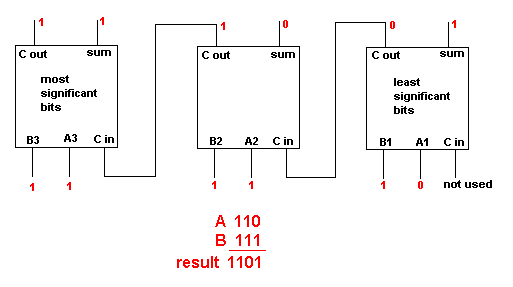Home > Electronics Tutorials > Combination Logic > 3 Bit Adder Tutorial

# Combination Logic Tutorial

The least significant bits (those on the right) are 0 and 1, giving a sum of 1 with no carry.
There is no carry in from a previous stage.

The next bits are 1 and 1 with no carry in, giving a sum of 0 and a carry of 1.

The most significant bits are both 1 with a carry in of 1.
This gives a sum of 1 and a carry of 1.

The result of adding 110 to 111 is 1101